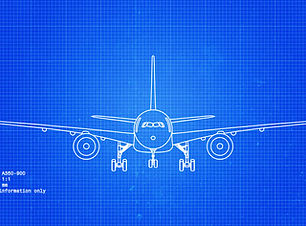DESCRIPTION:

This course builds the knowledge and competence of students regarding Arithmetic, Algebra and Geometry of Mathematics (Module 1) in accordance with EASA Part 66 categories B1 and B2 Basic knowledge level.

The course covers the elements of EASA Aviation Legislation as need by a Part 66 engineer including the structure of EASA rules, the role of the International Civil Aviation Organisation (ICAO), EASA and national CAAs.

This includes:

Detailed Content / Topics – The following Subjects will be addressed -

1. Arithmetic

1.1 Arithmetical terms and signs
1.2 Basic operations
1.3. Sequence of arithmetical operations
1.4. Factors and multiples
1.5. Fractions
1.7. Multiplication and division
1.8. Decimals
1.9. Weights, measures and conversion factors
1.10. Ratio and proportion
1.11. Powers
1.12. Averages
1.13. Percentages
1.14. Areas and volumes
1.15. Square and cube roots

2. Algebra

2.1. Simple algebraic expressions
2.2. Algebraic rules
2.3. Brackets
2.4. Equations
2.5. Linear equations
2.6. Equations containing simple fractions
2.7. Simultaneous equations
2.8. Indices and powers
2.9. Binary system
2.10. Second degree equations with one unknown
2.11. Logarithms

3. Geometry

3.1. Simple geometrical constructions
3.2. Graphical representation
3.3. Nature and use of graphs
3.4. Graphs and mathematical formulae
3.5. Simple trigonometry
3.6. Trigonometrically relationships
3.7. Co-ordinate geometry
3.8. Polar coordinates#### MATHEMATICS

EASA MODULE 1

Module 1, Mathematics focuses on examples covering Arithmetic, Algebra and Geometry.

Price.

£40

MATHEMATICS - MODULE 1 TRAINING

• This is an internet based training course for Mathematics (Arithmetic, Algebra, geometry).

• The course includes training notes, photographs, diagrams and a final multi-choice exam (pass mark 75%).

• On completion, you will be able to have your own serial numbered and recorded Training Certificate direct to your email (PDF format) and print a copy as required.